# Review of Significant Figures

Part of this lab is actually to demonstrate that significant figures are often NOT the best way to represent uncertainty. In general throughout this laboratory course, we will be using other techniques. However, to help you see the distinctions, we will ask you to use them in this lab. We assume that you are familiar with the concept of significant figures from other science courses. If you are not, however, the material below from OpenStax Chemistry 2e is provided for your reference.

The numbers of measured quantities, unlike defined or directly counted quantities, are not exact. To measure the volume of liquid in a graduated cylinder, you should make a reading at the bottom of the meniscus, the lowest point on the curved surface of the liquid. To measure the volume of liquid in this graduated cylinder, you must mentally subdivide the distance between the 21 and 22 mL marks into tenths of a milliliter, and then make a reading (estimate) at the bottom of the meniscus.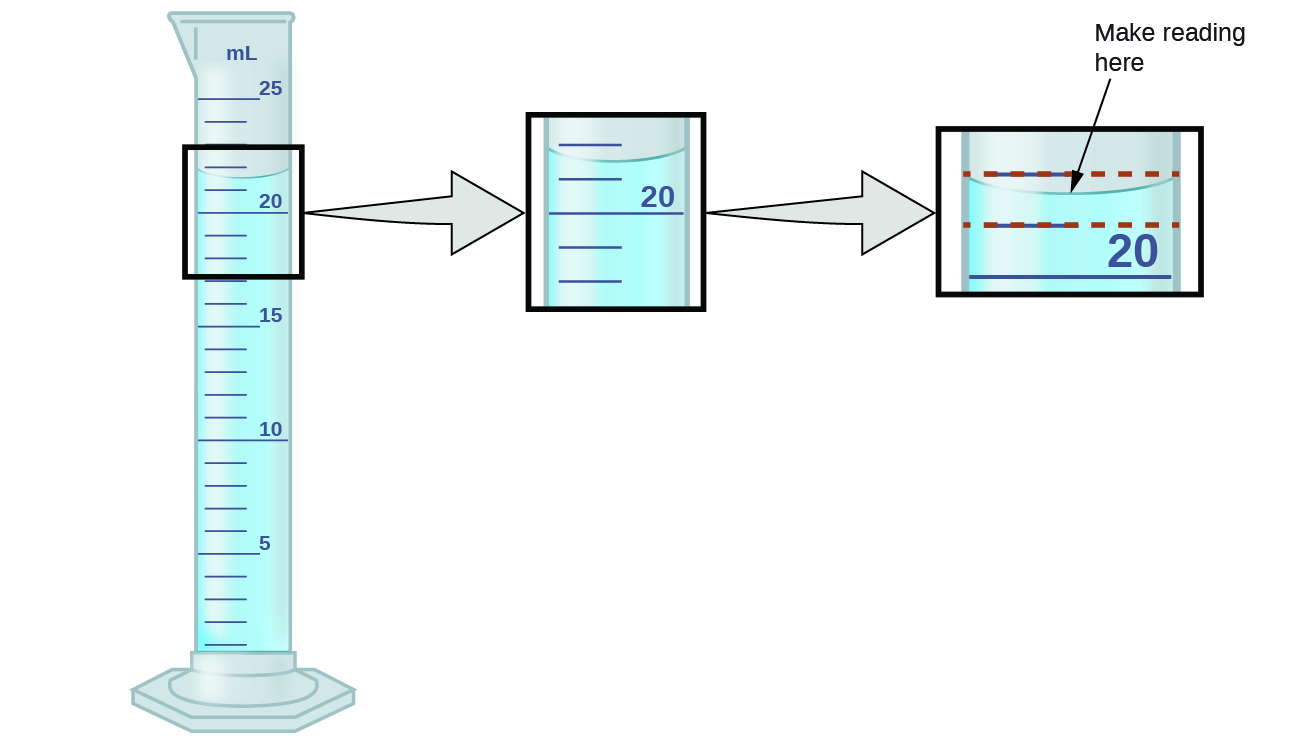Refer to the illustration in (Figure). The bottom of the meniscus in this case clearly lies between the 21 and 22 markings, meaning the liquid volume is certainly greater than 21 mL but less than 22 mL. The meniscus appears to be a bit closer to the 22-mL mark than to the 21-mL mark, and so a reasonable estimate of the liquid’s volume would be 21.6 mL. In the number 21.6, then, the digits 2 and 1 are certain, but the 6 is an estimate. Some people might estimate the meniscus position to be equally distant from each of the markings and estimate the tenth-place digit as 5, while others may think it to be even closer to the 22-mL mark and estimate this digit to be 7. Note that it would be pointless to attempt to estimate a digit for the hundredths place, given that the tenths-place digit is uncertain. In general, numerical scales such as the one on this graduated cylinder will permit measurements to one-tenth of the smallest scale division. The scale in this case has 1-mL divisions, and so volumes may be measured to the nearest 0.1 mL.

This concept holds true for all measurements, even if you do not actively make an estimate. If you place a quarter on a standard electronic balance, you may obtain a reading of 6.72 g. The digits 6 and 7 are certain, and the 2 indicates that the mass of the quarter is likely between 6.71 and 6.73 grams. The quarter weighs about 6.72 grams, with a nominal uncertainty in the measurement of ± 0.01 gram. If the coin is weighed on a more sensitive balance, the mass might be 6.723 g. This means its mass lies between 6.722 and 6.724 grams, an uncertainty of 0.001 gram. Every measurement has some uncertainty, which depends on the device used (and the user’s ability). All of the digits in a measurement, including the uncertain last digit, are called significant figures or significant digits. Note that zero may be a measured value; for example, if you stand on a scale that shows weight to the nearest pound and it shows “120,” then the 1 (hundreds), 2 (tens) and 0 (ones) are all significant (measured) values.

A measurement result is properly reported when its significant digits accurately represent the certainty of the measurement process. But what if you were analyzing a reported value and trying to determine what is significant and what is not? Well, for starters, all nonzero digits are significant, and it is only zeros that require some thought. We will use the terms “leading,” “trailing,” and “captive” for the zeros and will consider how to deal with them.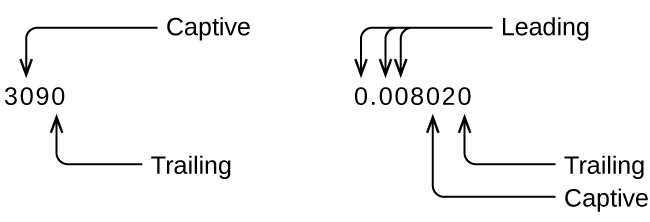Starting with the first nonzero digit on the left, count this digit and all remaining digits to the right. This is the number of significant figures in the measurement unless the last digit is a trailing zero lying to the left of the decimal point.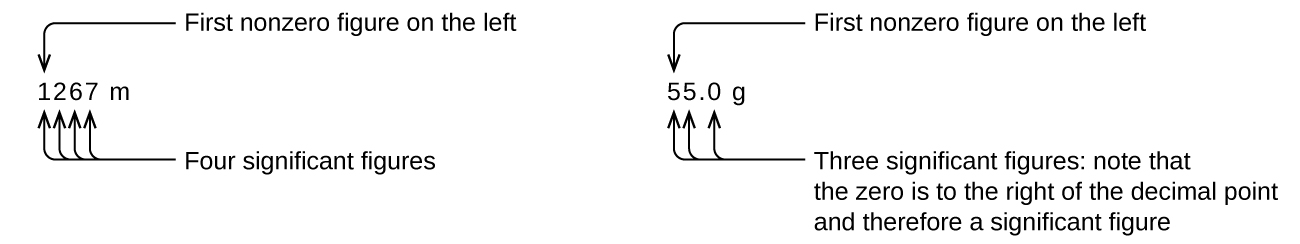Captive zeros result from measurement and are therefore always significant. Leading zeros, however, are never significant—they merely tell us where the decimal point is located.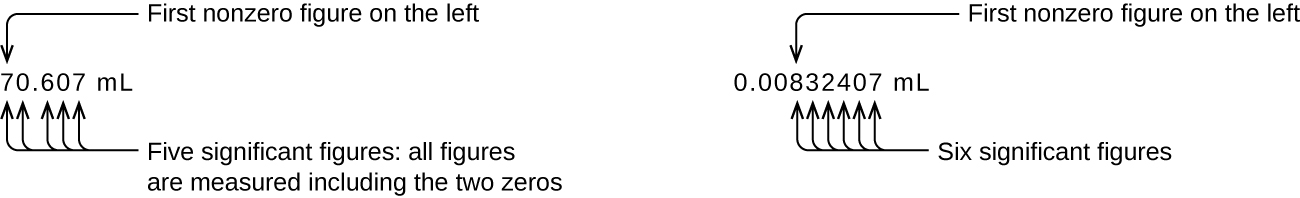The leading zeros in this example are not significant. We could use exponential notation (as described in Appendix B) and express the number as 8.3240710−3; then the number 8.32407 contains all of the significant figures, and 10−3 locates the decimal point.

The number of significant figures is uncertain in a number that ends with a zero to the left of the decimal point location. The zeros in the measurement 1,300 grams could be significant or they could simply indicate where the decimal point is located. The ambiguity can be resolved with the use of exponential notation: 1.3103 (two significant figures), 1.30103 (three significant figures, if the tens place was measured), or 1.300103 (four significant figures, if the ones place was also measured). In cases where only the decimal-formatted number is available, it is prudent to assume that all trailing zeros are not significant.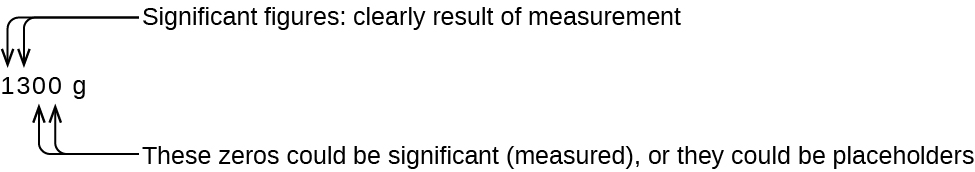When determining significant figures, be sure to pay attention to reported values and think about the measurement and significant figures in terms of what is reasonable or likely when evaluating whether the value makes sense. For example, the official January 2014 census reported the resident population of the US as 317,297,725. Do you think the US population was correctly determined to the reported nine significant figures, that is, to the exact number of people? People are constantly being born, dying, or moving into or out of the country, and assumptions are made to account for the large number of people who are not actually counted. Because of these uncertainties, it might be more reasonable to expect that we know the population to within perhaps a million or so, in which case the population should be reported as 3.17108 people.

### Significant Figures in Calculations

A second important principle of uncertainty is that results calculated from a measurement are at least as uncertain as the measurement itself. Take the uncertainty in measurements into account to avoid misrepresenting the uncertainty in calculated results. One way to do this is to report the result of a calculation with the correct number of significant figures, which is determined by the following three rules for rounding numbers:

1. When adding or subtracting numbers, round the result to the same number of decimal places as the number with the least number of decimal places (the least certain value in terms of addition and subtraction).
2. When multiplying or dividing numbers, round the result to the same number of digits as the number with the least number of significant figures (the least certain value in terms of multiplication and division).
3. If the digit to be dropped (the one immediately to the right of the digit to be retained) is less than 5, “round down” and leave the retained digit unchanged; if it is more than 5, “round up” and increase the retained digit by 1; if the dropped digit is 5, round up or down, whichever yields an even value for the retained digit. (The last part of this rule may strike you as a bit odd, but it’s based on reliable statistics and is aimed at avoiding any bias when dropping the digit “5,” since it is equally close to both possible values of the retained digit.)

The following examples illustrate the application of this rule in rounding a few different numbers to three significant figures:

• 0.028675 rounds “up” to 0.0287 (the dropped digit, 7, is greater than 5)
• 18.3384 rounds “down” to 18.3 (the dropped digit, 3, is less than 5)
• 6.8752 rounds “up” to 6.88 (the dropped digit is 5, and the retained digit is even)
• 92.85 rounds “down” to 92.8 (the dropped digit is 5, and the retained digit is even)

Let’s work through these rules with a few examples.

Rounding Numbers Round the following to the indicated number of significant figures:

(a) 31.57 (to two significant figures)

(b) 8.1649 (to three significant figures)

(c) 0.051065 (to four significant figures)

(d) 0.90275 (to four significant figures)

Solution (a) 31.57 rounds “up” to 32 (the dropped digit is 5, and the retained digit is even)

(b) 8.1649 rounds “down” to 8.16 (the dropped digit, 4, is less than 5)

(c) 0.051065 rounds “down” to 0.05106 (the dropped digit is 5, and the retained digit is even)

(d) 0.90275 rounds “up” to 0.9028 (the dropped digit is 5, and the retained digit is even)

Check Your Learning Round the following to the indicated number of significant figures:

(a) 0.424 (to two significant figures)

(b) 0.0038661 (to three significant figures)

(c) 421.25 (to four significant figures)

(d) 28,683.5 (to five significant figures)

(a) 0.42; (b) 0.00387; (c) 421.2; (d) 28,684

Addition and Subtraction with Significant Figures Rule: When adding or subtracting numbers, round the result to the same number of decimal places as the number with the fewest decimal places (i.e., the least certain value in terms of addition and subtraction).

(a) Add 1.0023 g and 4.383 g.

(b) Subtract 421.23 g from 486 g.

Solution

(a)Answer is 5.385 g (round to the thousandths place; three decimal places)

(b)Answer is 65 g (round to the ones place; no decimal places)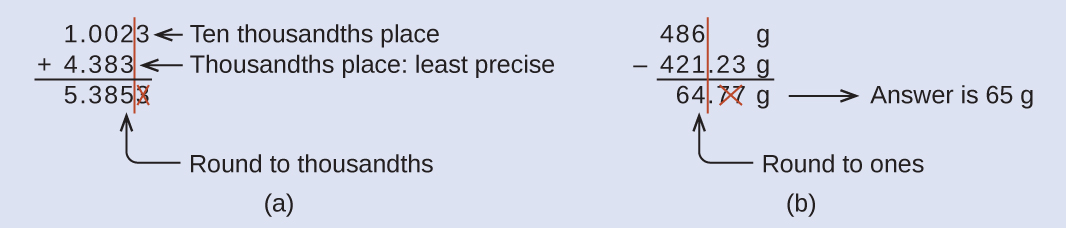(b) Subtract 55.8752 m from 56.533 m.

(a) 2.64 mL; (b) 0.658 m

Multiplication and Division with Significant Figures Rule: When multiplying or dividing numbers, round the result to the same number of digits as the number with the fewest significant figures (the least certain value in terms of multiplication and division).

(a) Multiply 0.6238 cm by 6.6 cm.

(b) Divide 421.23 g by 486 mL.

Solution

(a)(b)Check Your Learning (a) Multiply 2.334 cm and 0.320 cm.

(b) Divide 55.8752 m by 56.53 s.

(a) 0.747 cm2 (b) 0.9884 m/s

In the midst of all these technicalities, it is important to keep in mind the reason for these rules about significant figures and rounding—to correctly represent the certainty of the values reported and to ensure that a calculated result is not represented as being more certain than the least certain value used in the calculation.

Calculation with Significant Figures One common bathtub is 13.44 dm long, 5.920 dm wide, and 2.54 dm deep. Assume that the tub is rectangular and calculate its approximate volume in liters.

SolutionCheck Your Learning What is the density of a liquid with a mass of 31.1415 g and a volume of 30.13 cm3?

1.034 g/mL

Experimental Determination of Density Using Water Displacement A piece of rebar is weighed and then submerged in a graduated cylinder partially filled with water, with results as shown.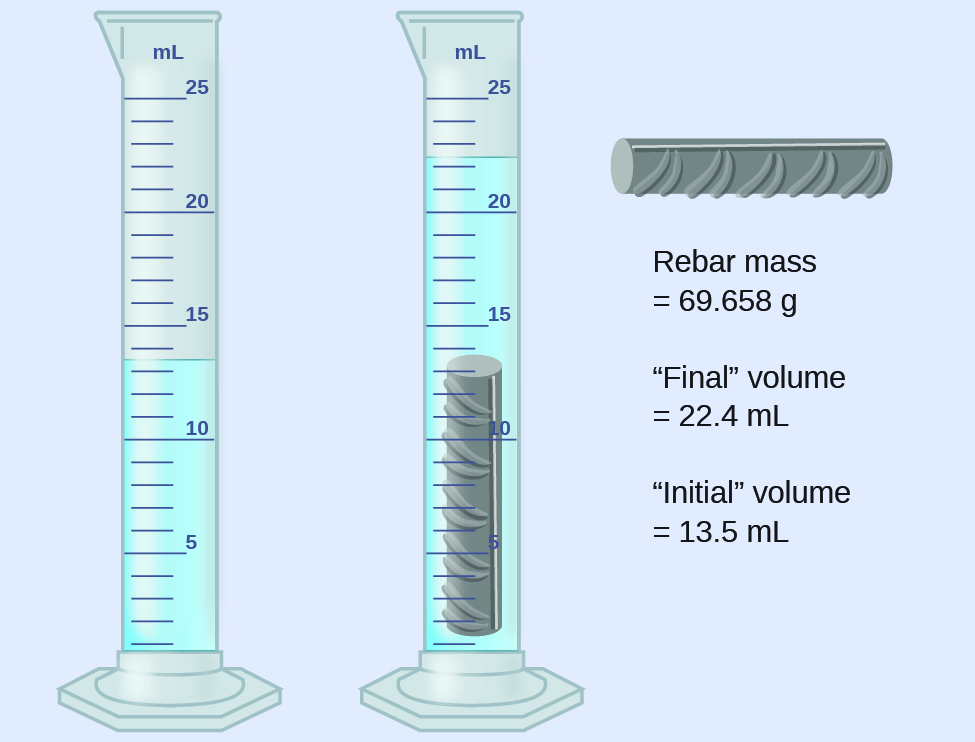(a) Use these values to determine the density of this piece of rebar.

(b) Rebar is mostly iron. Does your result in (a) support this statement? How?

SolutionThe volume of the piece of rebar is equal to the volume of the water displaced:(rounded to the nearest 0.1 mL, per the rule for addition and subtraction)

The density is the mass-to-volume ratio:(rounded to two significant figures, per the rule for multiplication and division)

From (Figure), the density of iron is 7.9 g/cm3, very close to that of rebar, which lends some support to the fact that rebar is mostly iron.

Check Your Learning An irregularly shaped piece of a shiny yellowish material is weighed and then submerged in a graduated cylinder, with results as shown.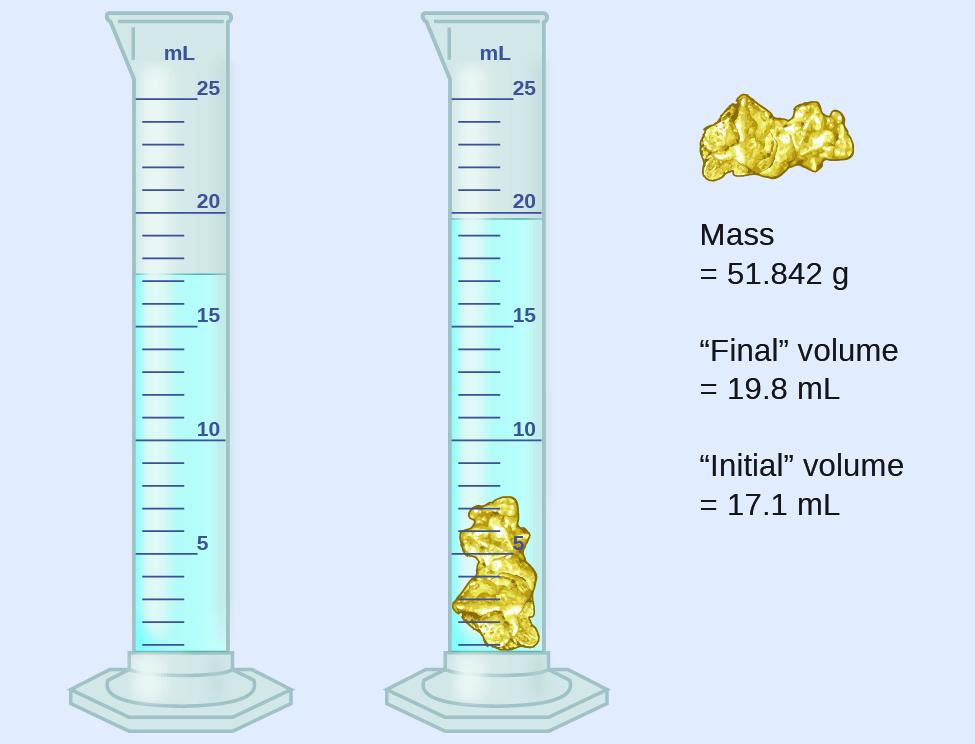(a) Use these values to determine the density of this material.

(b) Do you have any reasonable guesses as to the identity of this material? Explain your reasoning.